# ORF523: Mirror Descent, part I/II

In this post we are interested in minimizing a convex functionover a compact convex setunder the assumption thatis-Lipschitz onwith respect to some arbitrary norm(precisely we assume that). As we have seen in this post, using the correct norm to measure the variation of a function can make an enormous difference. We describe now a method invented by Nemirovski and Yudin to deal with this situation.

Let us for a moment abstract the situation a little bit and forget that we are trying to do optimization in finite dimension. We already observed that Projected Subgradient Descent works in an arbitrary Hilbert space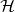(think of). However we are now interested in the more general situation where we are optimizing the function in some Banach space(for example). In that case the Gradient Descent strategy does not even make sense: indeed the gradients (more formally the Fréchet derivative)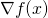are elements of the dual spaceand thus one cannot perform the computation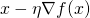(it simply does not make sense). We did not have this problem for optimization in an Hilbert spacesince by Riesz representation theorem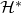is isometric to. The great insight of Nemirovski and Yudin is that one can still do a gradient descent by first mapping the pointinto the dual space, then performing the gradient update in the dual space, and finally mapping back the resulting point to the primal space. Of course the new point in the primal space might lie outside of the constraint setand thus we need a way to project back the point on the constraint set. The rest of this lecture will make this idea more precise.

Preliminaries

We consider a non-empty convex open setsuch that the constraint setis included in its closure, that is, and such that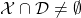.

Now we consider a continuously differentiable and strictly convex function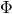defined on. This functionwill play the role of the mirror map between the primal and dual space. Precisely a pointin the primal is mapped toin the ‘dual’ (note that here all points lie in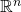and the notions of ‘primal’ and ‘dual’ space only have an intuitive meaning). In other words the gradient update of Mirror Descent with the mirror mapwill take the form. Next this dual point needs to be mapped back to the primal space, which is always possible under the condition that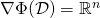(weaker assumptions are possible but we will not need them), that is one can findsuch that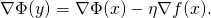Now this point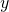might lie outside of the constraint set. To project back on the set of constraint we use the Bregman divergence associated todefined byThe projection ofontothen takes the following form:To ensure the existence of this projection we assume now thatsatisfiesWith this assumptionis locally increasing on the boundary of, which together with the fact thatis compact, andis stricly convex implies the existence and uniqueness of the projection defined above.

We say thatis a mirror map if it satisfies all the above assumptions.

Mirror Descent

We can now describe the Mirror Descent strategy based on a mirror map. Let. Then for, let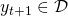such that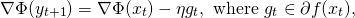andTheorem Letbe a mirror map. Assume also thatis strongly convex onwith respect to, that isLetandbe convex and-Lipschitz w.r.t., then Mirror Descent withsatisfiesProof: Let. The claimed bounded will be obtained by taking a limit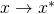. Now by convexity of, the definition of the Mirror Descent, and the definition of the Bregman divergence one hasNow the key observation is that by writing down the first order optimality condition forone obtains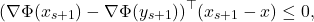which can be written equivalently as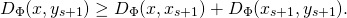Thus the term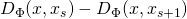will lead to a telescopic sum when summing overto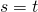, and it remains to bound the other term as follows: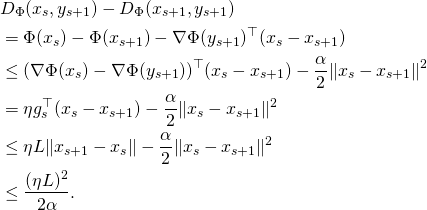We provedwhich concludes the proof up to trivial computation.Mirror Descent as a proximal method

One can rewrite Mirror Descent as follows:Nowadays this last expression is usually taken as the definition of Mirror Descent (see this paper by Beck and Teboulle). It gives a proximal point of view on Mirror Descent: the algorithm is trying to minimize the local linearization of the function while not moving too far away from the previous point (with distances measured via the Bregman divergence of the mirror map).

Next time we will see some of the ‘standard setups’ for Mirror Descent, that is pairs of (constraint set, mirror map) that ‘go well together’.

This entry was posted in Optimization. Bookmark the permalink.

## 7 Responses to "ORF523: Mirror Descent, part I/II"

•••••